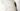# Angular Momentum of Which Set Of Quantum Numbers Cannot Specify An Orbital?When it comes to describing an electron in an atom, scientists use a set of quantum numbers. These numbers provide valuable information about the electron’s energy, position, and orientation within the atom. However, not all sets of quantum numbers can fully specify an orbital. In this article, we will explore the limitations of quantum numbers and identify which set is unable to provide a complete description of an orbital.

Quantum numbers play a crucial role in understanding the behavior of electrons in atoms. They help us determine the energy level, shape, and orientation of an electron’s orbital. However, there is one specific set of quantum numbers that falls short in providing a complete picture of an orbital. In this article, we will delve into the limitations of this particular set and explore why it cannot fully specify an orbital.

## Which Set Of Quantum Numbers Cannot Specify An Orbital?

Quantum numbers are a set of values used to describe the properties and behavior of electrons in an atom. They provide a way to understand the arrangement of electrons in an atom’s orbitals and their energy levels. By using quantum numbers, scientists can predict and explain the behavior of electrons in various chemical reactions and interactions.

There are four main quantum numbers:

1. Principal Quantum Number (n): This quantum number represents the energy level or shell in which an electron is located. It can have any positive integer value starting from 1. The higher the value of n, the higher the energy level and the farther the electron is from the nucleus.
2. Azimuthal Quantum Number (l): Also known as the orbital angular momentum quantum number, l determines the shape of the electron’s orbital. It can have values ranging from 0 to (n-1), where n is the principal quantum number. The values of l correspond to different subshells, such as s, p, d, and f.
3. Magnetic Quantum Number (ml): This quantum number describes the orientation of the orbital in space. It can have values ranging from -l to +l, representing the different orientations of the electron’s orbital within a particular subshell.
4. Spin Quantum Number (ms): The spin quantum number describes the spin of the electron. It can have two possible values: +1/2 (spin-up) or -1/2 (spin-down). This quantum number is related to the intrinsic angular momentum or spin of the electron.

These quantum numbers, when combined, provide a unique set of values that can be used to describe and differentiate each electron in an atom. However, while they are crucial for understanding the behavior of electrons, they do have limitations in fully specifying an electron’s orbital, as we will explore further in the next sections.

## Importance of Quantum Numbers in Specifying an Orbital

Quantum numbers play a crucial role in understanding the behavior and properties of electrons in an atom. They provide a framework for describing the arrangement and distribution of electrons in orbitals. However, there is a particular set of quantum numbers that falls short in fully specifying an orbital.

To comprehend why this limitation exists, we must first grasp the concept of quantum mechanics. In the realm of quantum mechanics, electrons are not simply tiny particles orbiting the nucleus in fixed paths, as classical physics would suggest. Instead, they exist in regions of space called orbitals, which are associated with different energy levels.

Quantum numbers are used to describe these orbitals and provide information about an electron’s energy, shape, orientation, and spin. The four main quantum numbers – principal quantum number (n), azimuthal quantum number (l), magnetic quantum number (ml), and spin quantum number (ms) – each contribute to specifying an electron’s orbital.